Sections in Howard Eves' Tetrahedron

Howard Eves describes a tetrahedron as follows:

... draw two line segments AB and CD perpendicular to one another, each of length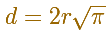and having the line segment joining their midpoints as a common perpendicular. "

The distance between these two lines is 2r.

This tetrahedron can be considered within a prism of a square base and height 2r.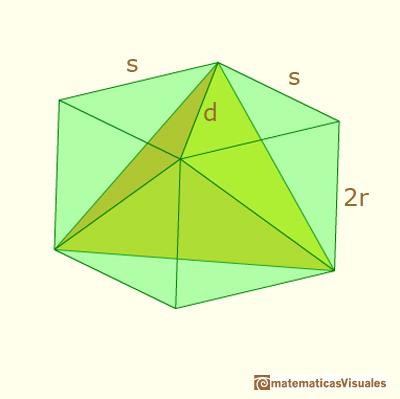The side of the square is

Therefore, the volume of the tetrahedron is

If x is the distance from the plane of the section that represents the applet to the center of the tetrahedron, the area of the section is (this is a particular case of sections of a tetrahedron):Now that we now how to calculate the volume of a tetrahedron and the cross-sections areas of a tetrahedron and a sphere we can see how Howard Eves mixed all these ideas to calculate the volume of a sphere using Cavalieri's Principle.

REFERENCES

Howard Eves, mathematician and historian of Mathematics, received the George Polya Award for the article Two Surprising Theorems on Cavalieri Congruence.The volume of a tetrahedron is one third of the prism that contains it.We want to calculate the surface area of sections of a sphere using the Pythagorean Theorem. We also study the relation with the Geometric Mean and the Right Triangle Altitude Theorem.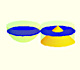Using Cavalieri's Principle we can calculate the volume of a sphere.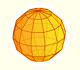We study a kind of polyhedra inscribed in a sphere, in particular the Campanus' sphere that was very popular during the Renaissance.We want to calculate the surface area of sections of a sphere using the Pythagorean Theorem. We also study the relation with the Geometric Mean and the Right Triangle Altitude Theorem.Leonardo da Vinci made several drawings of polyhedra for Luca Pacioli's book 'De divina proportione'. Here we can see an adaptation of the Campanus' sphere.Using Cavalieri's Principle we can calculate the volume of a sphere.Kepler used an intuitive infinitesimal approach to calculate the area of a circle.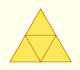The first drawing of a plane net of a regular tetrahedron was published by Dürer in his book 'Underweysung der Messung' ('Four Books of Measurement'), published in 1525 .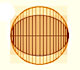In his book 'On Conoids and Spheroids', Archimedes calculated the area of an ellipse. We can see an intuitive approach to Archimedes' ideas.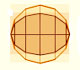In his book 'On Conoids and Spheroids', Archimedes calculated the area of an ellipse. It si a good example of a rigorous proof using a double reductio ad absurdum.Archimedes show us in 'The Method' how to use the lever law to discover the area of a parabolic segment.